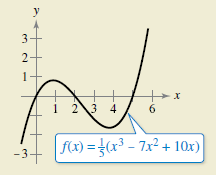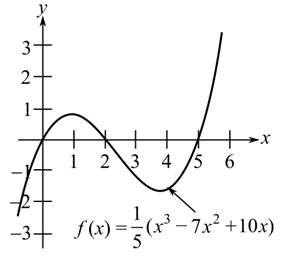Chapter 8.1, Problem 86E

Chapter
Section
Textbook Problem

HOE DO YOU SEE IT?Using the graph, is ∫ 0 5 f ( x )   d x positive or negative? Explain.To determine
If the integral 05f(x)dx is positive or negative where f(x) is shown in the graph as follows:Explanation

Since integration of a curve is the area under that curve, therefore, if the negative area is more under the curve than the positive area, then value of integration of f(x) is negative and if the positive area is more than the negative than the value of integral is positive

Still sussing out bartleby?

Check out a sample textbook solution.

See a sample solution

The Solution to Your Study Problems

Bartleby provides explanations to thousands of textbook problems written by our experts, many with advanced degrees!

Get Started

In Exercises 49-62, find the indicated limit, if it exists. 51. limx0x2xx

Applied Calculus for the Managerial, Life, and Social Sciences: A Brief Approach

In Problems 19-44, factor completely. 30.

Mathematical Applications for the Management, Life, and Social Sciences

Given: ABEFACDF12 Prove: BE

Elementary Geometry for College Students